Courses

# Chapter 4 Alternating Current Circuit - Notes, Circuit Theory, Electrical Engineering Electrical Engineering (EE) Notes | EduRev

## Electrical Engineering (EE) : Chapter 4 Alternating Current Circuit - Notes, Circuit Theory, Electrical Engineering Electrical Engineering (EE) Notes | EduRev

The document Chapter 4 Alternating Current Circuit - Notes, Circuit Theory, Electrical Engineering Electrical Engineering (EE) Notes | EduRev is a part of the Electrical Engineering (EE) Course Electrical Engineering SSC JE (Technical).
All you need of Electrical Engineering (EE) at this link: Electrical Engineering (EE)

Alternating Current Circuit

• Alternating Current (A.C) – The current whose magnitude and direction both change with time and the same cycle is repeated after a regular interval of time is called alternating current. In India its frequency is 50 Hz and its magnitude at time t is called instantaneous value and is given by
• I = I0 sin ωt
• where I0 = Peak value of current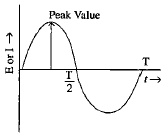As the variations are like sine curve, it is called sinusoidal A. C. Such type of A.C. is produced when a coil rotates in a uniform magnetic field with angular velocity ω. The flux associated with coil,
Ø = NBA cos ωt

where q = wt is the angle covered by normal to plane of coil with B-  in time t. Due to change in flux induced emf.
E = -df/dt = NBA w sinwt

E = Esin ωt and the corresponding current I = I0 sin ωt.

• Cycle – One complete set of positive and negative values of an alternating quantity is known as a cycle. A cycle may also sometimes be specified in terms of angular measure. In that case one complete cycle is said to spread over 360º or 2π radian.
• Amplitude :- The maximum value, positive or negative of an alternating quantity is known as its amplitude
•  Frequency (f):- The number of cycles/second is called the frequency of the alternating quantity.  Its unit is hertz (Hz).
• Time period (T) :- The time taken by an alternating quantity to complete the cycle is called its time period. Time period is the reciprocal of frequency.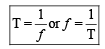• Root mean Square (R. M. S) Value :- The r.m.s. value of an alternating current is given by that steady (D.C.) current which when flowing through a given circuit for a given time produces the same heat as produced by the alternating current when flowing through the same circuit for the same time.

R.M.S. value is the value which is taken for power purposes of any description.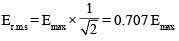• Average or mean value :- The average value of an alternating current is expressed by that steady current which transfers across any circuit the same change as is transferred by that alternating current during the same time.

Eav = 0.636 Emax 

• Form factor :- It is the ratio of R.M.S. value to the average value and denoted by Kf.

Form factor Kf  =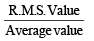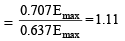Peak Value :- It is the ratio of max, value to R. M. S. Value or effective value. It is denoted by Kp.
Peak value Kp =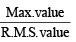S.NO. Wave form Form factor Kf Peak factor Kp 1. Sine wave –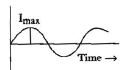R.M.S. value =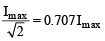Average value =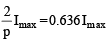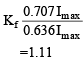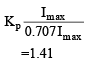2. Half wave rectified sine wave–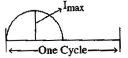R.M.S. value =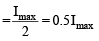Average value =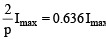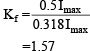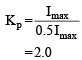3. Full wave rectified Sine wave –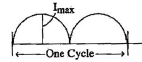R.M.S. value =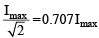average value =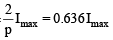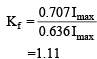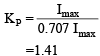4. Rectangular wave –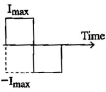R.M.S. value = Imax Average Value = Imax Kf = 1 KP = 1 5. Triangular wave –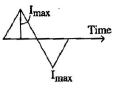R.M.S. value =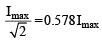Average value =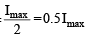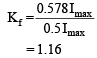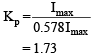Reasons for using alternating current (or voltage of sinusoidal form) –

(i) Mathematically it is quite simple.
(ii) Its integrals and differentials both are sinusoidal.
(iii) It tends itself to vector representation.
(iv) A complex wave form can be analysed into a series of sine waves of various frequencies and each such component can be dealt with separately.
(v) This wave form is desirable for power generation, transmission and utilisation.

A.C. Through pure Ohmic resistance alone – Where a sinusoidal e.m.f. is placed across a pure resistance the current will be in phase with thee.m.f. and it shown graphically will be in phase with the e.m.f. curve.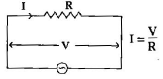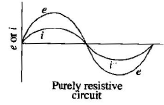The current I = V/R

A.C. Through pure capacitance alone – if a sinusoidal e.m.f. is placed across a capacitor the current will be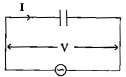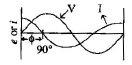I = (2πf)CV

f = frequency
V = Voltage (r.m.s. value)

Xc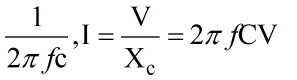The expression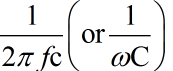is termed the capacitive reactance (XC) and the current is given by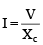. The power consumed is zero.

• Phasor Algebra – The following are the methods of representing vector quantities.

(i) Symbolic notation
(ii) Trigonometrical form
(iii) Exponential form
(iv) Polar form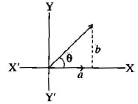(i) Symbolic notation E = a + jb

(ii) Trigonometrical form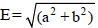(cosθ + jsinθ)

(iii) Exponential form E = [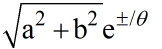general form]

(iv) Polar form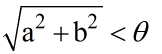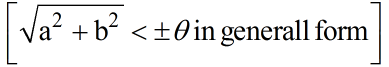• A. C. series circuits (R – L C ircuit Resistance and Inductance in series) –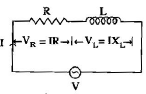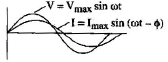formulae –

(1) Impedance Z =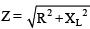(where XL = 2π  f LW)

(2) Current  I = V/Z

(3) Power factor cosø = R/Z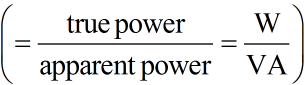(4) Power consumed P = VI cosø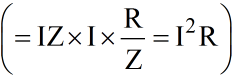R.C. circuit (Resistance and capacitance in series)

Formulae –

(1) Impedance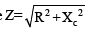(where Xc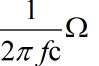)

(2) Current I = V/Z

(3) Power factor cosø = R/Z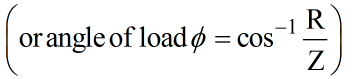(4) Power consumed = VI cosø( = I2P)

R-L-C circuit (Resistance, Inductance and capacitance in series)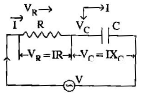Formulae –

(1) Impedance Z =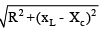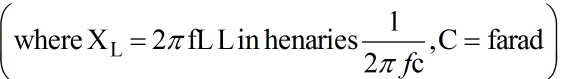(2) Current = I = V/Z

(3) Power factor cos ø = R/Z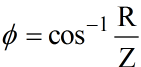(4) Power consumed = VI cos ø (= I2 R)

A. C. Parallel Circuit – When two or more branches are connected in parallel, the circuit is said to be parallel circuit.
Properties of parallel circuit:
(1) The voltage across each branch is same
(2) The current in each branch is different and depends upon the value of impedance.
(3) The angle between voltage and current of each branch will be different.
(4) The total current will be the vector sum of branch currents.
(5) The total impedance will be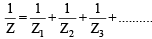(In A.C. series circuit Z = Z= Z+ Z3)

•  Comparison of series and parallel resonant circuit –
 S. No. Aspects Series circuit (R-L-c) Parallel circuit R-L andC 1. Impedance at resonance Minimum Maximum 2. Current at resonance Maximum V/R Minimum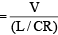3. Effective Impedance R L / CR 4. Power factor at resonance Unity Unity 5. Resonant frequency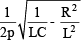6. It magnifies Voltage Current 7. Magnification is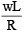• Three phase circuits – In case of three phase circuits, the three-phase currents are determined by considering each phase separately, and calculating the three-phase current from the phase voltage and impedances in the same manner as for single phase circuit. In practice three phase systems are usually symmetrical.

Having calculated the phase currents, the line currents are obtained from the following simple rule –

1. Star-connected system – Line current (IL) = phase current (Iph)

Line voltage (EL) = √3 phase voltage (= √3 Iph)

Line voltage (EL)= phase voltage (EPh)

2. Delta connected system

Line current (IL) = √3 phase current (= √3 Iph)

Line voltage (EL) = Phase voltage (Eph) 

Polyphase Circuits – More than one phase supply is called polyphase supply. If number of armature windings are used in the alternator, then this is called a polyphase alternator. The angle of displacement can be determined by the formula

Electrical displacement =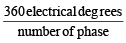Advantages of polyphase over single phase supply–
(i) The rating of a given machines increases with the increase in no. of phase. In case of three phase supply the rating of  3 phase motor will be nearly 1-5 times the output of single phase motor of same size.
(ii) For same ra ting the power factor of polyphase motor is higher than single phase motor
(iii) The efficiency of single phase motor is higher than polyphase motor.
(iv) In case of polyphase motors a revolving field is set up but in case of single phase motors there is no self starting.
(v) Polyphase system is more dependable than single phase system.
(vi) Parallel operation of 3 phase alternator is simple as compared to single phase alternator
(vii) The voltage regulation is less in polyphase system than single phase system
(viii) Polyphase motors are smooth in running than single phase motors.

Comparison between star and Delta connection
(i) In star connection similar ends are joined together where as in delta connection dissimilar ends are joined together.
(ii) In case of star connection, line voltage is √3 phase voltage whereas in case of delta connection line voltage is equal to phase voltage.

(iii) In case of star connection line current is equal to phase current whereas in delta connection line current is equal √3 phase current.
(iv) In case of star connection it is possible to carry the neutral wire to the load whereas in delta connection it is not possible.
(v) All distribution transformers are Delta/Star connected whereas power transformer are Delta/Delta connected.

(1) The generation of A.C. is found to be economical than that of D.C.
(2) The alternating voltage can be easily stepped up or stepped down by using a transformer.
(3) The alternating current can be regulated by using a choke coil without any significant wastage o electrical energy.
(4) The alternating voltage can be transmitted to distant places with only a very small loss in a.c. power.
(5) Further a.c. can be easily converted into d.c. by using rectifiers.

(1) The a.c. supply is mor e suidical and dangerous then d.c. in terms of shock received due to them.
(2) The alternating current always flows on the outer layer of the wire. It is called single thick wire used for d.c. the specially designed wire consisting of a number of thin wire is used.
(3) The alternating current can not be used in electrolytic processes such as electroplating, electrotyping etc.

Offer running on EduRev: Apply code STAYHOME200 to get INR 200 off on our premium plan EduRev Infinity!

65 docs|37 tests

,

,

,

,

,

,

,

,

,

,

,

,

,

,

,

,

,

,

,

,

,

,

,

,

,

,

,

;